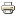# Design and operations of gas transmission networksWorking paper
Author/s:
Frédéric Babonneau, Yurii Nesterov and Jean-Philippe Vial
Issue number:
2009/48
Publisher:
CORE
Year:
2009
Problems dealing with the design and the operations of gas transmission networks are challenging. The difficulty mainly arises from the simultaneous modeling of gas transmission laws and of the investment costs. The combination of the two yields a non-linear non-convex optimization problem. To obviate this shortcoming, we propose a new formulation as a multi-objective problem, with two objectives. The first one is the investment cost function or a suitable approximation of it; the second is the cost of energy that is required to transmit the gas. This energy cost is approximated by the total energy dissipated into the network. This bi-criterion problem turns out to be convex and easily solvable by convex optimization solvers. Our continuous optimization formulation can be used as an efficient continuous relaxation for problems with non-divisible restrictions such as a limited number of available commercial pipe dimensions.
Developed by Paolo Gittoi